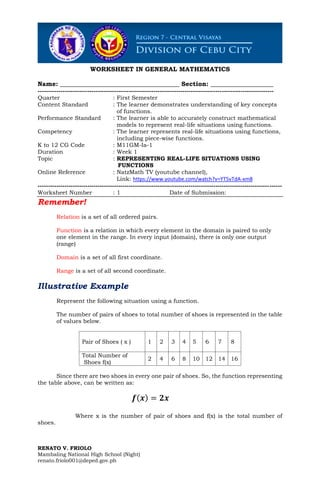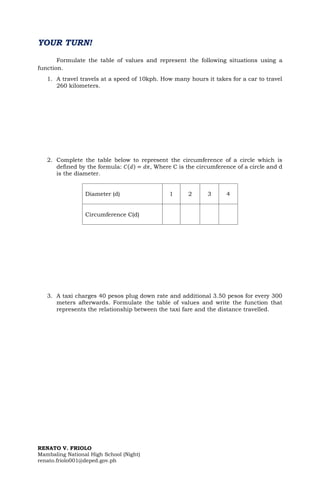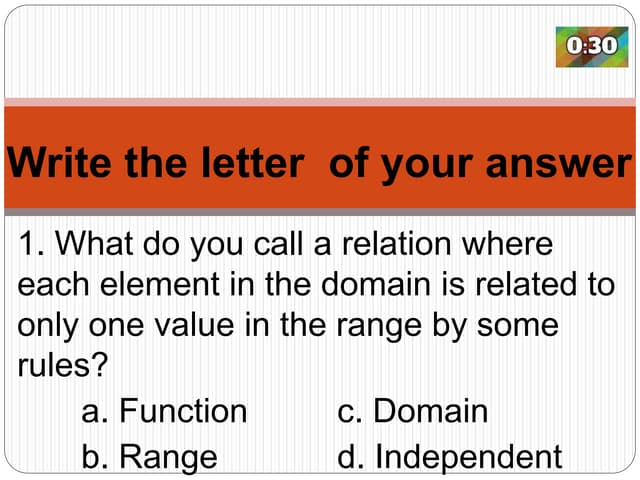PublicitéProchain SlideShareQUIZ 1.pptx
Chargement dans ... 3
1 sur 3
Publicité

### 1 Represent Real-Life Situations Using Functions.pdf

1. RENATO V. FRIOLO Mambaling National High School (Night) renato.friolo001@deped.gov.ph WORKSHEET IN GENERAL MATHEMATICS Name: ______________________________________ Section: ____________________ ------------------------------------------------------------------------------------------------------------------ Quarter : First Semester Content Standard : The learner demonstrates understanding of key concepts of functions. Performance Standard : The learner is able to accurately construct mathematical models to represent real-life situations using functions. Competency : The learner represents real-life situations using functions, including piece-wise functions. K to 12 CG Code : M11GM-Ia-1 Duration : Week 1 Topic : REPRESENTING REAL-LIFE SITUATIONS USING FUNCTIONS Online Reference : NatzMath TV (youtube channel), Link: https://www.youtube.com/watch?v=YT5vTdA-xm8 ---------------------------------------------------------------------------------------------------------------------- Worksheet Number : 1 Date of Submission: Remember! Relation is a set of all ordered pairs. Function is a relation in which every element in the domain is paired to only one element in the range. In every input (domain), there is only one output (range) Domain is a set of all first coordinate. Range is a set of all second coordinate. Illustrative Example Represent the following situation using a function. The number of pairs of shoes to total number of shoes is represented in the table of values below. Pair of Shoes ( x ) 1 2 3 4 5 6 7 8 Total Number of Shoes f(x) 2 4 6 8 10 12 14 16 Since there are two shoes in every one pair of shoes. So, the function representing the table above, can be written as: 𝒇(𝒙) = 𝟐𝒙 Where x is the number of pair of shoes and f(x) is the total number of shoes.
2. RENATO V. FRIOLO Mambaling National High School (Night) renato.friolo001@deped.gov.ph YOUR TURN! Formulate the table of values and represent the following situations using a function. 1. A travel travels at a speed of 10kph. How many hours it takes for a car to travel 260 kilometers. 2. Complete the table below to represent the circumference of a circle which is defined by the formula: 𝐶(𝑑) = 𝑑𝜋, Where C is the circumference of a circle and d is the diameter. Diameter (d) 1 2 3 4 Circumference C(d) 3. A taxi charges 40 pesos plug down rate and additional 3.50 pesos for every 300 meters afterwards. Formulate the table of values and write the function that represents the relationship between the taxi fare and the distance travelled.
3. RENATO V. FRIOLO Mambaling National High School (Night) renato.friolo001@deped.gov.ph
Publicité